# Design and Analyze High Pass Filter using pcbComponent

This example shows how to design and analyze high pass filter using the pcbComponent object. A microstrip high pass filter is designed based on a three-pole (n = 3) Chebyshev high pass prototype with 0.1 dB passband ripple and cut-off frequency ${f}_{c}$ = 1.5 GHz.

### Design and Analyze High Pass Filter

The element values of the corresponding low pass Chebyshev prototype are taken from Table 3.2 of reference 

• ${g}_{0}$ = ${g}_{4}$ = 1.0

• ${g}_{1}$ = ${g}_{3}$ = 1.0316

• ${g}_{2}$ = 1.1474

Using the design equations Eqs. (6.2) and (6.3) given in reference  for n = 3 and ${Z}_{0}$ = 50 ohm terminations, we can obtain quasi-lumped circuit elements values as

• ${C}_{1}$ = ${C}_{3}$ = 2.0571 pF

• ${L}_{2}$ = 4.6236 nH

A schematic diagram of such a high pass filter taken from fig 6.2 of reference  representing various feature dimensions is shown below. It is seen that the series capacitors ${C}_{1}$ and ${C}_{3}$ are realized by identical interdigital capacitors, while the shunt inductor ${L}_{2}$ is realized by a short-circuited stub. A commercial substrate (`RT/D 5880`) having a relative dielectric constant of 2.2 and thickness of 1.57 mm is chosen for this microstrip filter realization. The dimensions of the interdigital capacitors, such as the finger length, width, spacing between fingers, and number of the fingers are given in the fig 6.2 of reference . The procedure to calculate the dimensions of interdigital capacitor and stub inductor is discussed in the section 6.1.1 of reference . A closed-form design formulation for interdigital capacitor and alternatively full-wave EM simulations are used to extract desired values of lumped circult capacitance for different feature dimensions.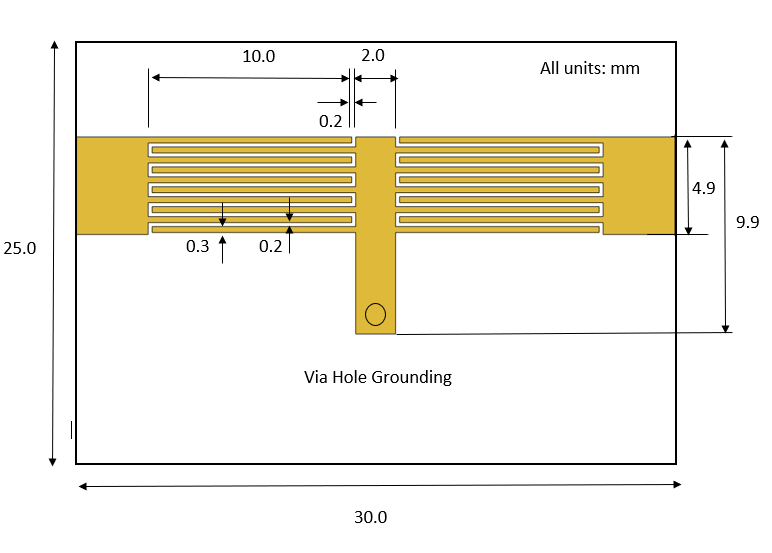Use the `interdigitalCapacitor` object and change its properties as per given values in reference  to create interdigited fingers. Visualize created object using `show`

```idc = interdigitalCapacitor; idc.NumFingers = 10; idc.FingerLength = 10e-3; idc.FingerWidth = 0.3e-3; idc.FingerSpacing = 0.2e-3; idc.FingerEdgeGap = 0.2e-3; idc.GroundPlaneWidth = 5e-3; idc.TerminalStripWidth = 0.1e-3; idc.PortLineLength = 0.1e-3; idc.PortLineWidth = 4.8e-3; figure; show(idc);```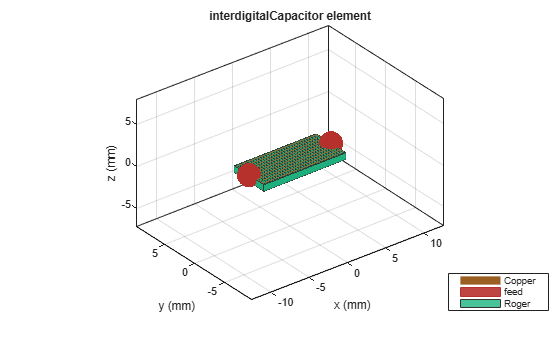Use the` pcbComponent` on `idc` object to create a capacitor `cap1`. Visualize `cap1` using `show`

```pcb = pcbComponent(idc); cap1 = pcb.Layers{1}; figure; show(cap1);```Use the `copy`, `rotateZ, rotateX` and `translate` operation methods on capacitor `cap1` object to create capacior `cap2`. Visualize `cap2` using `show`

```cap2 = copy(cap1); cap2 = rotateZ(cap2,180); cap2 = rotateX(cap2,180); cap2 = translate(cap2,[-6.2e-3 0 0]); cap1 = translate(cap1,[6.2e-3 0 0]); figure; show(cap2);```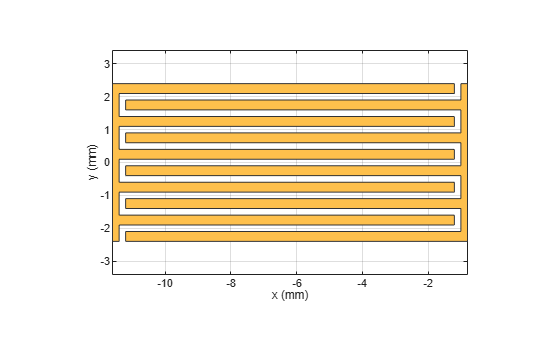Use the `traceRectangular` object to create both feeding ports `port1`, `port2` and short circuited stub `centerArm`. Perform a Boolean add operation for the shapes `port1`, `cap2`, `centerArm`, `cap1` and `port2` to create `filter`. Visualize `filter` using `show`

```portW = 4.9e-3; portL = 3.6e-3; centerL = 2e-3; centerW = 9.9e-3; port1 = traceRectangular(Length = portL,Width = portW,Center = [-11.4e-3-portL/2 -0.05e-3]); port2 = traceRectangular(Length = portL,Width = portW,Center = [11.4e-3+portL/2 -0.05e-3]); centerArm = traceRectangular(Length = centerL,Width = centerW,Center = [0 -2.55e-3]); filter = port1 + cap2 + centerArm + cap1 + port2; figure; show(filter);```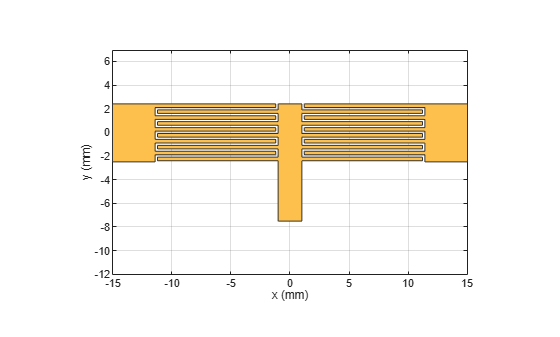Define the substrate parameters and create a dielectric to use in the `pcbComponent` of the designed filter. Create a groundplane using the `traceRectangular` shape.

Use the `pcbComponent` to create a filter PCB. Assign the dielectric and ground plane to the `Layers` property on `pcbComponent`. Assign the `FeedLocations` to the edge of the feed ports. Assign `ViaLocations` at the edge of stub `centerArm`. Set the `BoardThickness` to 1.57 mm on the `pcbComponent` and visualize the filter. The below code performs these operations and creates the filter `pcb` object.

```substrate = dielectric(EpsilonR = 2.2, LossTangent = 0.0009,... Name = "custom", Thickness = 1.57e-3); gndL = 30e-3; gndW = 25e-3; ground = traceRectangular(Length = gndL, Width = gndW,... Center = [0,-4e-3]); pcb = pcbComponent; pcb.BoardShape = ground; pcb.BoardThickness = 1.57e-3; pcb.Layers ={filter,substrate,ground}; pcb.FeedDiameter = portW/2; pcb.FeedLocations = [-gndL/2 0 1 3;gndL/2 0 1 3]; pcb.ViaDiameter = centerL; pcb.ViaLocations = [0 -6.5e-3 1 3]; figure; show(pcb);```Use the `sparameters` method to calculate the S-parameters for the high pass filter and plot it using the `rfplot` function.

```spar = sparameters(pcb,linspace(0.1e9,3.5e9,40)); figure; rfplot(spar);```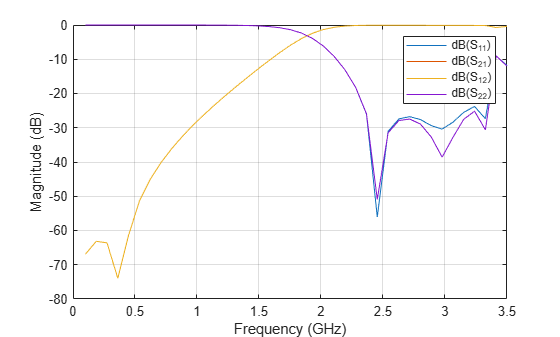As there are four curves in the result, let us analyze the results.

### Plot S-Parameters

Analyze the values of ${S}_{11}$, and ${S}_{12}$ to understand the behavior of high pass filter.

```figure; rfplot(spar,1,1); hold on; rfplot(spar,1,2); hold on;```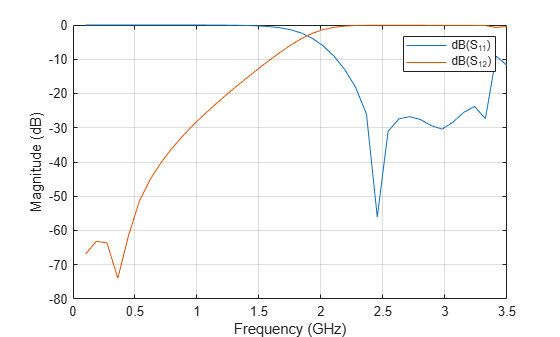The result shows that the filter has -3 dB cut-off frequency ${f}_{c}$ = 1.8 GHz . The ${S}_{12}$ values are greater than -3 dB and ${S}_{11}$ values are less than -10 dB above -3 dB cut-off frequency 1.8 GHz. The designed filter therefore has high pass response and em simulated results matches closely with reference. The 0.3 GHz shift in -3 dB cut-off frequency might be due to use of different numerical solver.

Use the `charge` method to visualize the charge distribution on the metal surface and dielectric of high pass filter

```figure; charge(pcb,3e9);``````figure; charge(pcb,3e9,'dielectric');```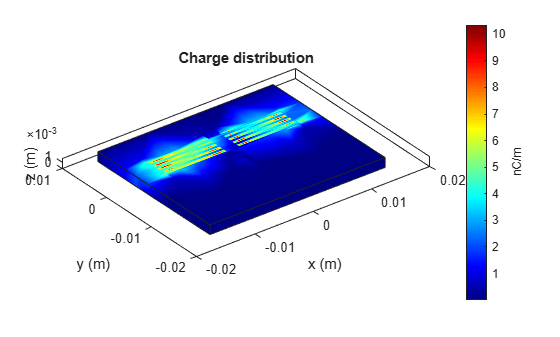Use the `current` method to visualize the current distribution on the metal surface and volume polarization currents on dielectric of high pass filter

```figure; current(pcb,3e9);```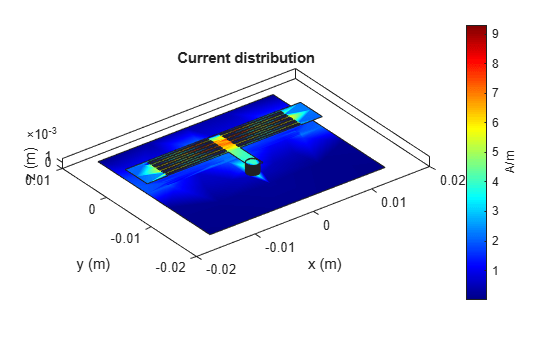```figure; current(pcb,3e9,'dielectric');```### References

 Jia-Sheng Hong "Microstrip Filters for RF/Microwave Applications", p. 165, John Wiley & Sons, 2nd Edition, 2011.